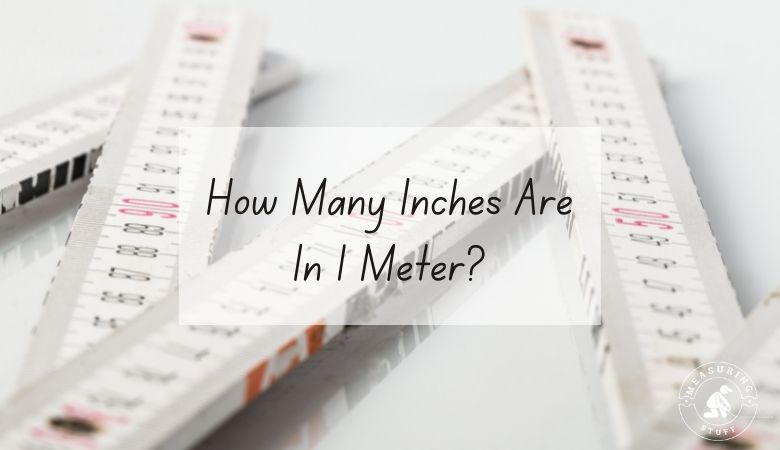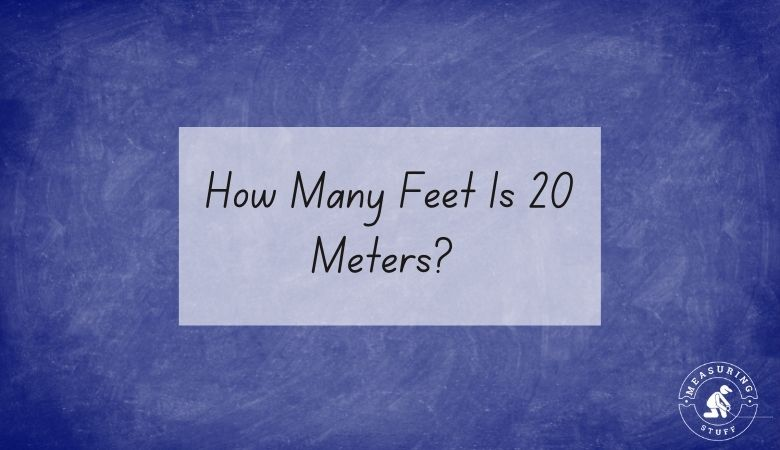# Length/Distance## How Many Inches Are In 1 Meter?

Meters and inches are units of measurement for distance, height, or length. Meters are used in the metric system, while inches are part of the imperial system. Because they’re part of two different systems, it can sometimes be confusing to try to convert between the two. A simple mathematical equation, however, can help. There are …## How Many Feet Is 20 Meters? (Easy Conversion Method)

20 meters equals 65.617 feet. To convert 20 meters into feet, you need to multiply by the conversion factor of 3.281. For example, use the following formula and multiply the number of meters by 3.281 to get the answer in feet. 20 meters x 3.281 (conversion factor) = 65.617 feet. This means that there are …